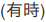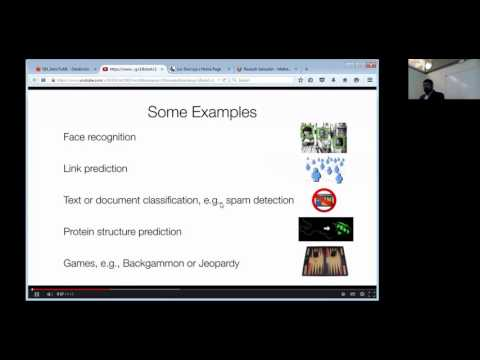// Databricks notebook source exported at Sat, 18 Jun 2016 11:01:37 UTC

# Scalable Data Science

### prepared by Raazesh Sainudiin and Sivanand Sivaram

The html source url of this databricks notebook and its recorded Uji:SOURCE: This is the scala version of the python notebook from the databricks Community Edition that has been added to this databricks shard at Workspace -> scalable-data-science -> xtraResources -> dbCE -> MLlib -> unsupervised -> clustering -> k-means -> 1MSongsPy_ETLExploreModel as extra resources for this project-focussed course Scalable Data Science.

# Stage 2: Exploring songs dataThis is the second notebook in this tutorial. In this notebook we do what any data scientist does with their data right after parsing it: exploring and understanding different aspect of data. Make sure you understand how we get the `songsTable` by reading and running the ETL notebook. In the ETL notebook we created and cached a temporary table named `songsTable`

## A first inspection

A first step to any data exploration is viewing sample data. For this purpose we can use a simple SQL query that returns first 10 rows.

``````
%sql select * from songsTable limit 10

``````
``````
table("songsTable").printSchema()

``````
``````
%sql select count(*) from songsTable

``````
``````
table("songsTable").count() // or equivalently with DataFrame API - recall table("songsTable") is a DataFrame

``````
``````
display(sqlContext.sql("SELECT duration, year FROM songsTable")) // Aggregation is set to 'Average' in 'Plot Options'

``````

## Exercises

1. Why do you think average song durations increase dramatically in 70’s?
2. Add error bars with standard deviation around each average point in the plot.
3. How did average loudness change over time?
4. How did tempo change over time?
5. What other aspects of songs can you explore with this technique?

## Sampling and visualizing

Another technique for visually exploring large data, which we are going to try, is sampling data.

• First step is generating a sample.
• With sampled data we can produce a scatter plot as follows.
``````
%python
# let's use ggplot from python
from ggplot import *
sampled = sqlContext.sql("select year, duration from songsTable where year > 1930 and year < 2012")\
.sample(withReplacement = False, fraction = 0.1).toPandas()

p = ggplot(sampled, aes(x = 'year', y = 'duration')) + ylim(0, 800) + \
geom_smooth(size=3, span=0.3) + geom_point(aes(color = 'blue', size = 4))
display(p)

``````

## Exercises

1. Add jitter to year value in the plot above.
2. Plot sampled points for other parameters in the data.

Next step is clustering the data. Click on the next notebook (Model) to follow the tutorial.

Updated: